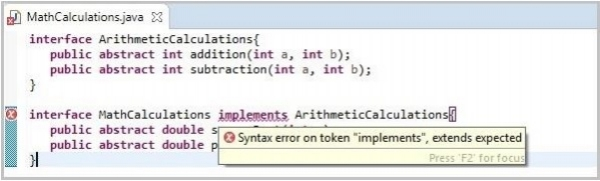# Can we implement one interface from another in java?

Java 8Object Oriented ProgrammingProgramming

No, we cannot implement one interface from another you can just extend it using the extends keyword as −

interface ArithmeticCalculations{
public abstract int addition(int a, int b);
public abstract int subtraction(int a, int b);
}
interface MathCalculations implements ArithmeticCalculations{
public abstract double squareRoot(int a);
public abstract double powerOf(int a, int b);
}

Still, if you try to implement one interface from another using the implements keyword. The compiler does not recognize the implements keyword after the name of the interface and throws a compile time error saying “'{' expected”.

## Example

In the following Java program, we have two interfaces namely, ArithmeticCalculations and MathCalculations and, we are trying to implement on interface from the other.

interface ArithmeticCalculations{
public abstract int addition(int a, int b);
public abstract int subtraction(int a, int b);
}
interface MathCalculations implements ArithmeticCalculations{
public abstract double squareRoot(int a);
public abstract double powerOf(int a, int b);
}

## Compile time error

On compiling, the above program generates the following compile time error −

## Output

MathCalculations.java:8: error: '{' expected
interface MathCalculations implements ArithmeticCalculations{
^
1 error

If you compile the same program using eclipse it gives you a compile time error suggesting that “extends keyword is expected instead of implements”.